# Broken Sticks, Triangles, and Probability II

Last week, we looked at two solutions to the problem of finding the probability that you can make a triangle using three pieces of a stick, if we cut it at two independently chosen, random locations. This time, we look another solution to that problem, and a similar solution to the version in which we choose the locations of the cuts one at a time. This will also give us a good opportunity to see how errors happen in probability problems,

## Two cuts at once

In 2007 we got this question yet again:

Three Pieces of a Stick Forming a Triangle

If you break a straight stick into three pieces, what is the probability that you can join the pieces end-to-end to form a triangle?

No specific method of breaking is stated here. Doctor Greenie answered, assuming that two locations are chosen randomly:

Hi, Bob -

After seeing your message, I sat down and figured out what I thought was a good answer.  Then I used Google to search for "break a stick into three pieces" and found several links which gave contradictory answers.  Many of the sites, however, showed the same answer I got, which is 1/4.

You can try a search of the web yourself to try to find information on this topic.  All of the formal and informal arguments I found on any of the pages I visited were far more complicated than the method I used to get my answer....

The contradictory answers presumably were for different interpretations of the random breaking, though perhaps some were just errors. And you may find that what follows looks more complicated than our others, but the underlying idea is very simple and elegant.

Here is the key idea for my analysis of the problem:

If we consider the original stick to be of unit length, then we can form a triangle whenever the longest stick is less than a half unit long.

This idea is based on the Triangle Inequality Theorem, which says that in a triangle the sum of any two sides must be greater than the third side.  Having one piece that is equal to or more than half a unit would leave the other two pieces to sum to exactly or less than half a unit, violating the theorem.

This should have been obvious! If the total length is 1, and one piece were longer than $$\frac{1}{2}$$, then the sum of the other two would be less than $$\frac{1}{2}$$, and therefore less than the longest piece – so there would be no triangle. And in fact, the inequalities we got in the first method last week were $$y-x<\frac{1}{2}\\x<\frac{1}{2}\\y>\frac{1}{2}$$ which essentially say that the three pieces, $$x$$, $$y-x$$, and $$1-y$$, are all less than $$\frac{1}{2}$$.

For my analysis, we consider different cases where we make the first break and then, based on where that first break is, determine where the second break can be in order for the three resulting pieces to be of lengths such that they can form a triangle.  So imagine the original stick marked like a ruler from 0.000 to 1.000.  Pick a few points for the first break and determine the range where the second break can be made so that the three pieces can form a triangle.  This determination is based solely on the key idea stated above.

Here is a sample first cut: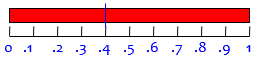Where could the second cut be in order to make a triangle? Give it a little thought yourself, then continue.

Our first case is when $$x< \frac{1}{2}$$:

Suppose we make the first break at 0.4.  We must make the second break somewhere after 0.5, because the "last" piece can't be more than a half unit long.  And for the "middle" piece to be less than a half unit long, we need to make the second break before 0.9 (that is, before (0.4 + 0.5).  So, for a first break at 0.4, the range over which we can make the second break is from 0.5 to 0.9, which is a range of 0.4.

If you pick any other point "x" less than 0.5 for the first break, then you will get a similar analysis; the second break will then have to be made between 0.5 and (x + 0.5), which is a range of x.

Here is this example; the green zone shows what locations for the second cut, y, are acceptable, and the four examples below it show one case in each red zone and two in the green zone, to make it clear what goes wrong, or doesn’t: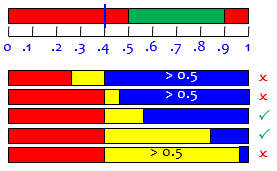We see that the second cut can’t be to the left of the first, because we’d be left with more than half of the stick in the right piece. And it must be between 0.5 to the right of the left end, and 0.5 to the left of the right end.

We conclude that, if the first cut is at $$x< \frac{1}{2}$$, then the probability of being able to make a triangle after the second cut is $$x$$.

Now let's pick a first break at a point past 0.5; suppose we make it at 0.85.  Then we will need to make the second break before 0.5 (so that the "first" piece is not more than a half unit long); and we will need to make the second break no farther than a half unit from the first break--i.e., at a point no less than 0.35.  So for a first break at 0.85, the range where we can make the second break and get three pieces which can form a triangle is from 0.35 to 0.5--a range of 0.15.

If you pick any other point "x" greater than 0.5 for the first break, then you will get a similar analysis; the second break will then have to be made between (x - 0.5) and 0.5, which is a range of (1 - x).

Here is this example, similar to the image above: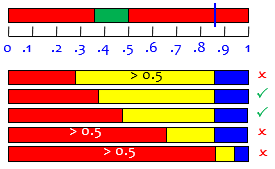We conclude that, if the first cut is at $$x> \frac{1}{2}$$, then the probability of being able to make a triangle after the second cut is $$1-x$$.

Now if we make a graph of the range of values for which the second break can be made as a function of where the first break is made, the graph increases uniformly from a value of 0 at x=0 to a value of 0.5 at a value of x=0.5; then it decreases uniformly to a value of 0 at x=1.0.  By a simple geometric argument, the average value of that graph over the entire interval is 1/4; therefore, the probability that the three pieces of the stick will form a triangle is 1/4.

The function, formally, is $$P(x)=\left\{\begin{matrix}x & \text{if } x\le\frac{1}{2}\\ 1-x & \text{if } x>\frac{1}{2}\end{matrix}\right.$$

Here is the graph: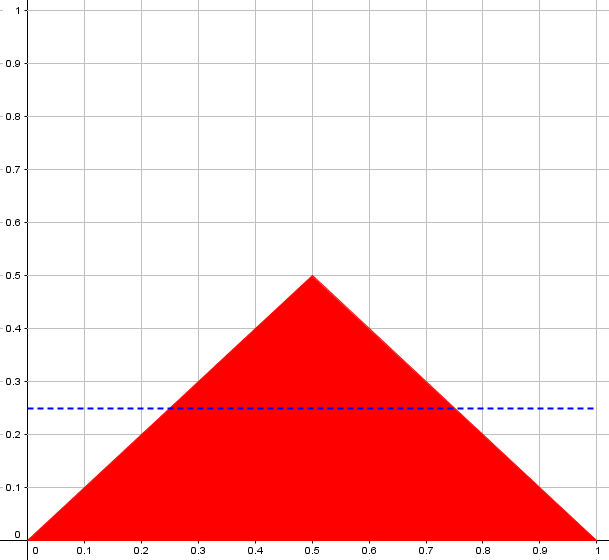The average probability, over all first cuts, is the blue line, at $$\frac{1}{4}$$. And if you compare this to the graph from the first method, you’ll see that all the red pixels have just fallen in a heap on the bottom; the area is the same.

## Cut once, then cut the larger piece

The next month, still in 2007, we got a question explicitly about a different version of the problem:

Probability of Making Triangle with Three Stick Pieces

Take a stick of unit length and break it into two pieces, choosing the break point at random.  Now break the longer of the two pieces at a random point.  What is the probability that the three pieces can be used to form a triangle?

I'm not sure what to do after taking the initial condition.  Let A be the point of breakage and its distance from one end is x.  Then x > 1/2.  The second probability gives y > 1/4.  How to proceed from here and get the probability?

Doctor Greenie answered this one, too:

Hello, Kiran -

I made a rather extensive search of the Internet and the Dr. Math archives and found no satisfactory discussion of this problem.  There are several sites I found which discuss the similar problem where the stick is broken randomly in two places--unlike your problem, where the first break is made and then the second break is made in the longer piece.  Even in that other case, I found disagreement about the correct answer.  And all of the sites I found used methods of solving the problem which seem more difficult than mine.

He starts with a fact he explained last time:

So here is my solution to your problem....

To make a triangle out of three pieces of a stick of unit length, the longest piece must have a length which is less than half a unit.

Let's make our first break and let x be the length of the shorter piece.  x can vary between 0 and 0.5.  Let's pick values in this range and consider the probability that we can make a triangle for that value of x.

Suppose x = 0.2; then the long stick has length 0.8.  The break on this stick must be made so that the longest piece has length less than 0.5.  This means the break must be made between 0.3 and 0.5.  So the probability of being able to make a triangle with the three pieces if the first break is at 0.2 is 0.2/0.8.

Rather than take x specifically as the location of the first cut, he takes it as the length of the shorter piece from that first cut. If we took x as the location, as before, we could replace x in what follows with $$\min(x, 1-x)$$, and let x run from 0 to 1 rather than from 0 to $$\frac{1}{2}$$.

Here is a picture of this first example; I’ve put the length x at the right this time, so that our second cut can be anywhere to its left, but in order to make a triangle, it must be in the green zone: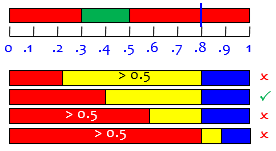Because the green zone has width $$x=0.2$$, out of a total possible range of 0.8, the probability of making a triangle given this first cut is $$\frac{0.2}{0.8}=0.25$$.

Now let's pick one more value for where we make the first break.  Suppose x = 0.4; then the long stick has length 0.6.  Again, the break on this stick must be made so that the longest piece has length less than 0.5.  This means the break must be made between 0.1 and 0.5.  So the probability of being able to make a triangle with the three pieces if the first break is at 0.4 is 0.4/0.6.

Here is a picture of this second example: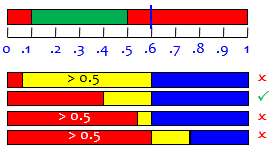Because the green zone has width $$x=0.4$$, out of a total possible range of 0.6, the probability of making a triangle given this first cut is $$\frac{0.4}{0.6}=0.\overline{6}$$.

In general, the green zone has width x, out of a possible region of 1 – x, so the probability of a triangle is $$\frac{x}{1-x}$$.

Using our two specific examples, we can see that, in the general case, the probability of being able to make a triangle out of the three pieces, as a function of the length x of the short piece we get on our first break, is

x/(1 - x)

Our variable x can range from 0 to 0.5, so the overall probability that we can make a triangle with the three pieces of the stick, given your statement of the problem, is

integral from 0 to 0.5 of (x/(1 - x))
---------------------------------------
0.5 - 0

This turns out to be

2(-.5 - ln(.5)) = 2(.19314718....) = .38629436...

The integral is the calculus way to find the area, as in the first answer above we found the area geometrically. The concept is identical in both cases, though we couldn’t keep it geometrical this time.

Here is a graph of the probability, if we take x as position and allow it to vary from 0 to 1 (to make it more like the graph in the previous problem); Doctor Greenie’s version is just the left half of this, which doesn’t affect the result: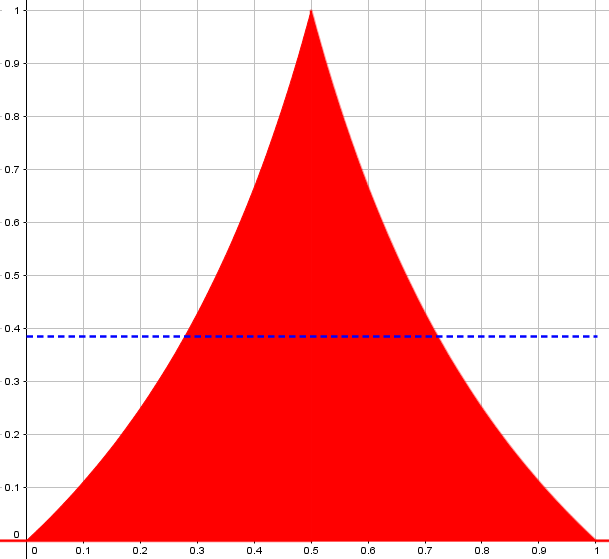The integral he did is not hard: $$\int\frac{x}{1-x}dx=\int\left(-1+\frac{1}{1-x}\right)dx=-x-\ln|1-x|+C$$ so that $$\int_0^{0.5}\frac{x}{1-x}dx=\left[-x-\ln|1-x|\right]_0^{0.5}=-0.5-\ln(0.5)\approx 0.38629436$$ The probability can be simplified to $$2\ln(2)-1$$.

## Tracking down errors

If you read the original page, you’ll see that my name is included at the bottom. That is because there was an error in the original answer, which gave half the correct answer (by failing to divide by 0.5); in 2008, and again twice in 2014, people wrote to us telling about simulations they had done that contradicted the answer, some pointing out the cause of the error. What you see above is the correct version that I proposed.

The last of these was particularly interesting. Abhigyan had read Doctor Greenie’s first answer in this post, giving the answer 0.25, and said this:

I see your answer to this problem beautifully described as compared to other solutions on Google search.

Satisfaction is one thing and verification is another. So I ran an Excel simulation to conclude that the probability turns out to be approximately 19.3% and not 1/4 or 25%.

With 10000 data points, the realization was ~20% and with 1000000 data points the realization was ~19.3%, giving me all the more reason to believe that the answer should not approach 25%.

I don't understand how mathematics can go wrong. In fact, I do not believe it. But I am trying to understand what is the correct answer to the problem (with or without mathematics)

So his result agreed with Doctor Greenie’s original answer to the second question, though he thought he was simulating the first! He offered to send me his spreadsheet; I replied:

This kind of problem requires a clear definition of the process that produces the results whose probability you are finding; the page you refer to does not specifically define how the breaks are to be made (though we can infer that from the work), so it is quite likely that your simulation is for a subtly different problem. I'll want to look at that when I see the file.

We have answered this kind of question many times, in different ways. Here are the answers I find, which will help you see the subtlety:

Break a dowel to form a triangle [states two options]
http://mathforum.org/library/drmath/view/54754.html

Probability of a Triangle [answer 1/4]
http://mathforum.org/library/drmath/view/56579.html

Probability of Forming a Triangle [answer 1/4]
http://mathforum.org/library/drmath/view/52203.html

Three Pieces of a Stick Forming a Triangle [the one you read]
http://mathforum.org/library/drmath/view/70224.html

Probability of Making Triangle with Three Stick Pieces [0.193]
http://mathforum.org/library/drmath/view/70602.html

The last answer deals with the alternative method of breaking the stick that is mentioned in the first, and gives an answer that agrees perfectly with your experimental data; I strongly suspect that you have use that method of breaking the stick in your simulation!

When he sent his spreadsheets (one with result 25% and the other 19.3%, with a comment that I was right about his using a different scheme originally), I looked into it more deeply and wrote back:

Those look good. But one thing makes me curious: the version of the problem for which the answer was 19.3% involved making the second break randomly in the LONGER piece left by the first break; but your second simulation makes the second break randomly in the RIGHTMOST piece left by the first break, without determining which is longer. As a result, I would expect this to give too small a probability, since half the time it is dividing the smaller piece, which can't produce a triangle.

I modified the simulation to make the first number always between 0 and 1/2, which is equivalent to taking it as the smaller of the two pieces (which is exactly what Dr. Greenie did in his work). This gives a probability of 38.59%.

Looking into this, I see that Dr. Greenie's work is wrong. In fact, someone wrote us in 2008 saying their simulation gave 38.6%, and someone else wrote us just this April saying the same thing. At that time I wrote up a request for our archivist to change the page.

I had totally forgotten this, though I in fact did my own simulation at the time, confirming the claim. I will have to expedite the correction.

Abhigyan’s original version (19.3%) was in fact doing the equivalent of Doctor Steve’s first interpretation from last week’s post, randomly choosing which half to break. And that was the problem that Doctor Greenie accidentally answered.

This demonstrates how easy it is to misinterpret a problem like this, even when you are coding a simulation to actually carry out the process. This is why I particularly like solving any probability problem in two ways before committing myself to the answer!

### 2 thoughts on “Broken Sticks, Triangles, and Probability II”

1.This would be the part where I, somewhat embarrassedly, point out that I wrote that. Michael Lugo Oct 23 ’09 at 4:13 It remains to note that those lines create inside the big triangle a small 578 triangle which is similar to big but with all lengths

1.I don’t understand what you are saying. None of this is relevant to this post.

This site uses Akismet to reduce spam. Learn how your comment data is processed.